## Cual es el modulo del vector diferencia (a – b) entre dos vectores que forman un angulo de 30° entre si y cuyos módulos son 2m y 8m. Grafica

Question

Cual es el modulo del vector diferencia (a – b) entre dos vectores que forman un angulo de 30° entre si y cuyos módulos son 2m y 8m. Grafica (Les agradecería muchísimo si me ayudan)

in progress 0
3 months 2021-07-29T14:25:05+00:00 1 Answers 5 views 0

|a-b|=6.34m

Explanation:

To find the difference between the vector you can use the formulas for the magnitude of a vector and also for the doc product between two vectors: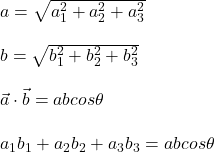a1, a2, a3: components of a vector

b1, b2, b3: components of b vector

a: magnitude of a = 2m

b: magnitude of b = 8m

angle = 30°

By squaring the first two equations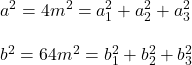Then, you multiply by 2 the third equation: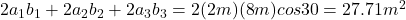Now, you sum the first two equations ans take the difference with the third equation. Thus, you obtain a perfect square trinomial: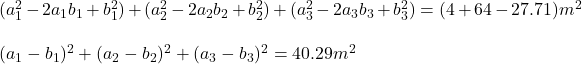This last expression is the square of the magnitude of the difference a-b. Hence you have: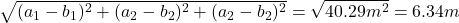thus, the magnitude of the difference is 6.34m